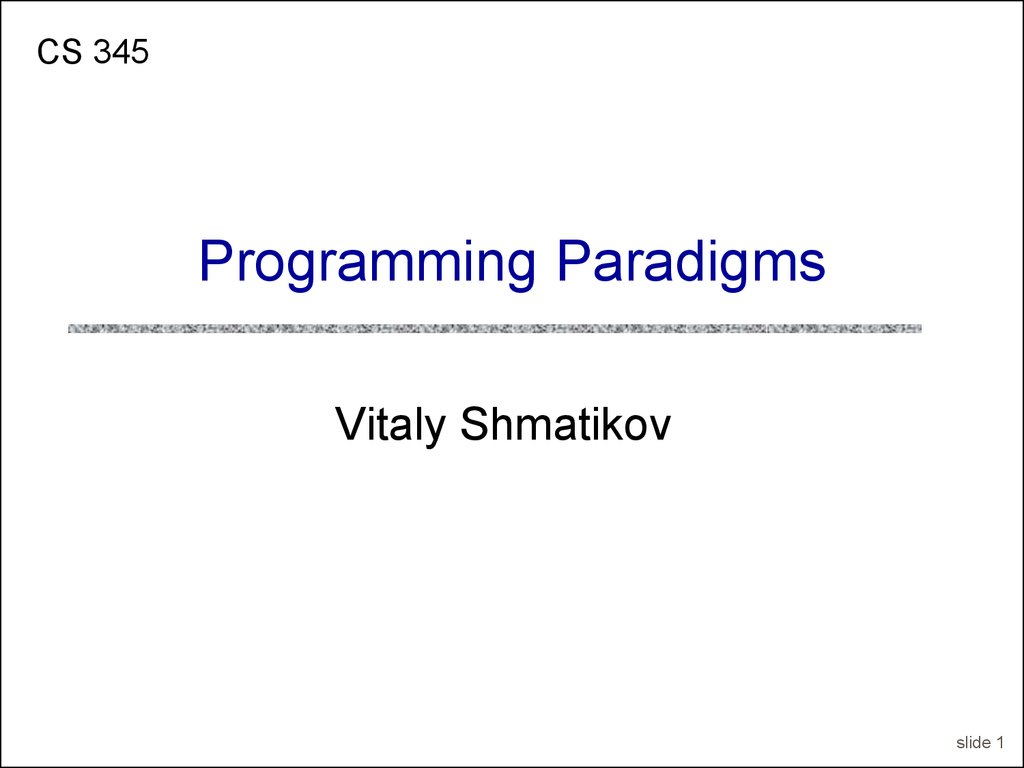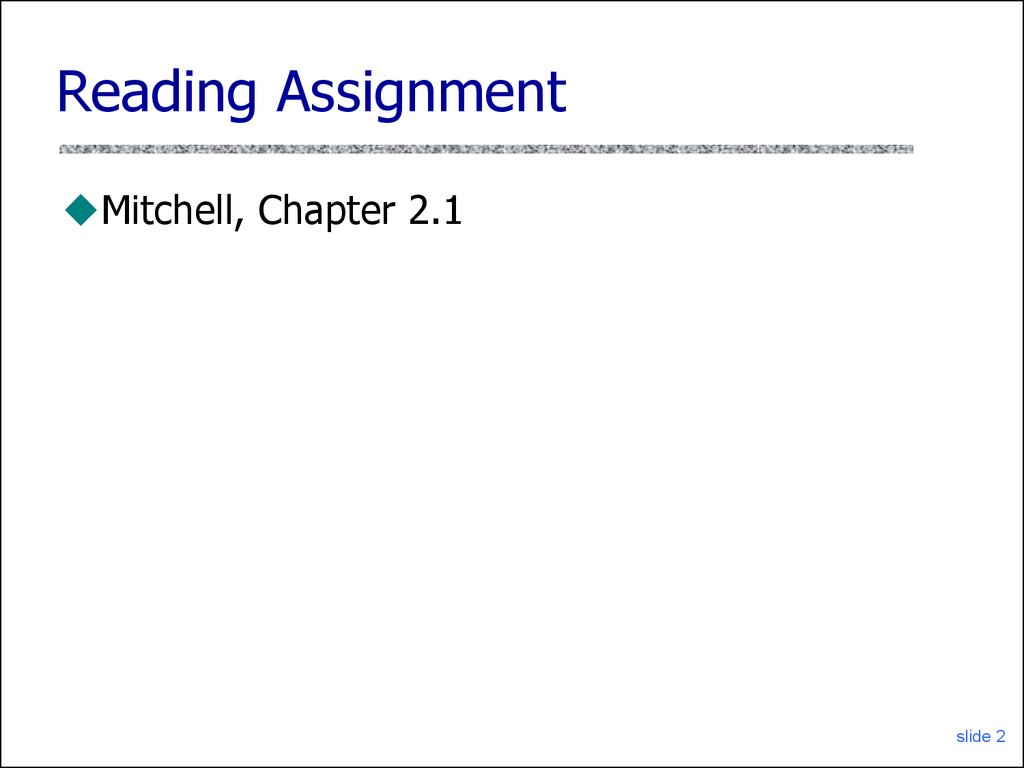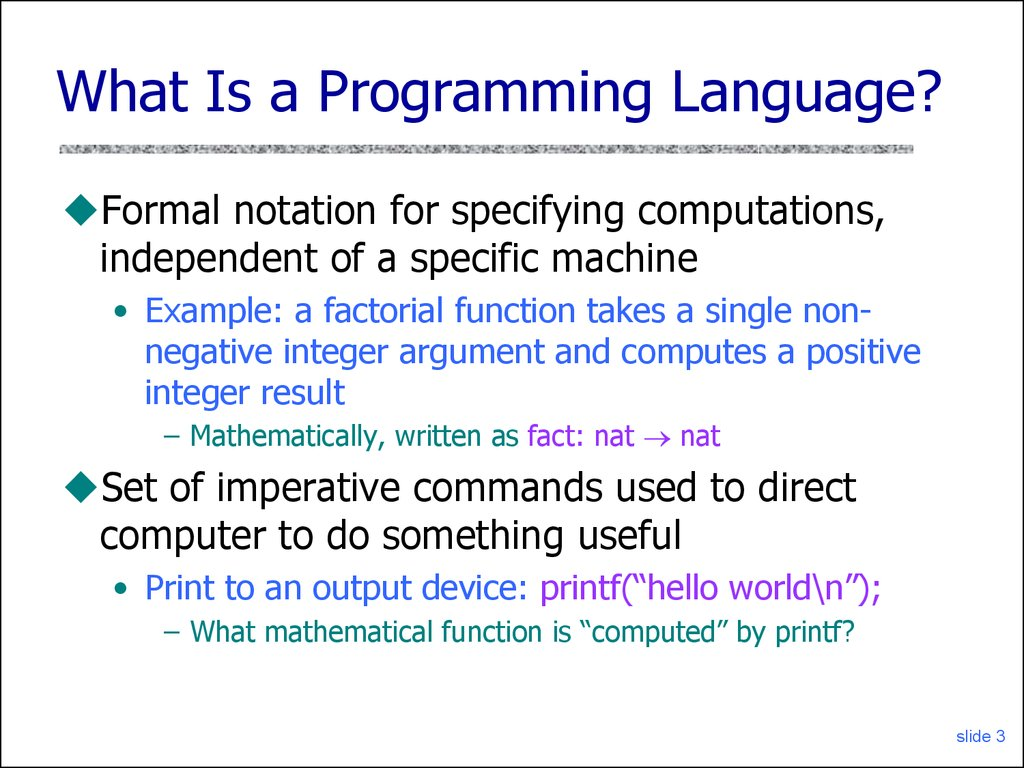CS 345
Vitaly Shmatikov
slide 1

Mitchell, Chapter 2.1
slide 2

## 3. What Is a Programming Language?

Formal notation for specifying computations,
independent of a specific machine
• Example: a factorial function takes a single nonnegative integer argument and computes a positive
integer result
– Mathematically, written as fact: nat nat
Set of imperative commands used to direct
computer to do something useful
• Print to an output device: printf(“hello world\n”);
– What mathematical function is “computed” by printf?
slide 3

## 4. Partial and Total Functions

Value of an expression may be undefined
• Undefined operation, e.g., division by zero
– 3/0 has no value
– Implementation may halt with error condition
• Nontermination
– f(x) = if x=0 then 1 else f(x-2)
– This is a partial function: not defined on all arguments
– Cannot be detected by inspecting expression (why?)
These two cases are “mathematically”
equivalent, but operationally different (why?)
Subtle: “undefined” is not the name of a function value …
slide 4

## 5. Partial and Total: Definitions

Total function f:A B is a subset f A B with
• x A, there is some y B with x,y f
(total)
• If x,y f and x,z f then y=z
(single-valued)
Partial function f:A B is a subset f A B with
• If x,y f and x,z f then y=z
(single-valued)
Programs define partial functions for two reasons
• What are these reasons?
slide 5

## 6. Computability

Function f is computable if some program P
computes it
• For any input x, the computation P(x) halts with
output f(x)
• Partial recursive functions: partial functions (int to
int) that are computable
slide 6

## 7. Halting Problem

Ettore Bugatti: "I make my
cars to go, not to stop"
slide 7

## 8. Halting Function

Decide whether program halts on input
• Given program P and input x to P,
Halt(P,x) =
yes if P(x) halts
no otherwise
Clarifications
• Assume program P requires one string input x
• Write P(x) for output of P when run in input x
• Program P is string input to Halt
Fact: There is no program for Halt
slide 8

## 9. Unsolvability of the Halting Problem

Suppose P solves variant of halting problem
yes if Q(Q) halts
• On input Q, assume P(Q) =
no otherwise
Build program D
• D(Q) =
run forever
halt
if Q(Q) halts
if Q(Q) runs forever
If D(D) halts, then D(D) runs forever
If D(D) runs forever, then D(D) halts
slide 9

## 10. Main Points About Computability

Some functions are computable, some are not
• Example: halting problem
Programming language implementation
an undefined basic operation (e.g., division by zero)
slide 10

## 11. Computation Rules

The factorial function type declaration does not
convey how the computation is to proceed
We also need a computation rule
• fact (0) = 1
• fact (n) = n * fact(n-1)
This notation is more computationally oriented
and can almost be executed by a machine
slide 11

## 12. Factorial Functions

C, C++, Java:
int fact (int n) { return (n == 0) ? 1 : n * fact (n-1); }
Scheme:
(define fact
(lambda (n) (if (= n 0) 1 (* n (fact (- n 1))))))
ML:
fun fact n = if n=0 then 1 else n*fact(n-1);
• fact :: Integer->Integer
• fact 0 = 1
• fact n = n*fact(n-1)
slide 12

Imperative / Procedural
Functional / Applicative
Object-Oriented
Concurrent
Logic
Scripting
In reality, very few languages are “pure”
• Most combine features of different paradigms
slide 13

## 14. Where Do Paradigms Come From?

Paradigms emerge as the result of social
processes in which people develop ideas
and create principles and practices that embody
those ideas
• Thomas Kuhn. “The Structure of Scientific Revolutions.”
Programming paradigms are the result of people’s
ideas about how programs should be constructed
• … and formal linguistic mechanisms for expressing them
• … and software engineering principles and practices for
using the resulting programming language to solve
problems
slide 14

Imperative (procedural) programs consists of
actions to effect state change, principally through
assignment operations or side effects
• Fortran, Algol, Cobol, PL/I, Pascal, Modula-2, Ada, C
• Why does imperative programming dominate in
practice?
OO programming is not always imperative, but
most OO languages have been imperative
• Simula, Smalltalk, C++, Modula-3, Java
• Notable exception: CLOS (Common Lisp Object System)
slide 15

## 16. Functional and Logic Paradigms

Focuses on function evaluation; avoids updates,
assignment, mutable state, side effects
Not all functional languages are “pure”
• In practice, rely on non-pure functions for input/output
and some permit assignment-like operators
– E.g., (set! x 1) in Scheme
Logic programming is based on predicate logic
• Targeted at theorem-proving languages, automated
reasoning, database applications
• Recent trend: declarative programming
slide 16

## 17. Concurrent and Scripting Languages

Concurrent programming cuts across imperative,
Scripting is a very “high” level of programming
• Rapid development; glue together different programs
• Often dynamically typed, with only int, float, string, and
array as the data types; no user-defined types
• Weakly typed: a variable ‘x’ can be assigned a value of
any type at any time during execution
Very popular in Web development
• Especially scripting active Web pages
slide 17

## 18. Unifying Concepts

Unifying language concepts
• Types (both built-in and user-defined)
– Specify constraints on functions and data
– Static vs. dynamic typing
• Expressions (e.g., arithmetic, boolean, strings)
• Functions/procedures
• Commands
We will study how these are defined syntactically,
used semantically, and implemented pragmatically
slide 18

## 19. Design Choices

C: Efficient imperative programming with static types
C++: Object-oriented programming with static types and
ad hoc, subtype and parametric polymorphism
Java: Imperative, object-oriented, and concurrent
programming with static types and garbage collection
Scheme: Lexically scoped, applicative-style recursive
programming with dynamic types
Standard ML: Practical functional programming with strict
(eager) evaluation and polymorphic type inference
Haskell: Pure functional programming with non-strict (lazy)
evaluation.
slide 19

## 20. Abstraction and Modularization

Re-use, sharing, extension of code are critically
important in software engineering
Big idea: detect errors at compile-time, not when
program is executed
Type definitions and declarations
• Define intent for both functions/procedures and data
Lexical scope
slide 20

## 21. Static vs. Dynamic Typing

Static typing
• Common in compiled languages, considered “safer”
• Type of each variable determined at compile-time;
constrains the set of values it can hold at run-time
Dynamic typing
• Common in interpreted languages
• Types are associated with a variable at run-time; may
change dynamically to conform to the type of the value
currently referenced by the variable
• Type errors not detected until a piece of code is
executed
slide 21

## 22. Billion-Dollar Mistake

Failed launch of Ariane 5 rocket (1996)
• \$500 million payload; \$7 billion spent on development
Cause: software error in inertial reference system
• Re-used Ariane 4 code, but flight path was different
• 64-bit floating point number related to horizontal
velocity converted to 16-bit signed integer; the number
was larger than 32,767; inertial guidance crashed
slide 22

## 23. Program Correctness

Assert formal correctness statements about critical
parts of a program and reason effectively
• A program is intended to carry out a specific
computation, but a programmer can fail to adequately
address all data value ranges, input conditions, system
resource constraints, memory limitations, etc.
Language features and their interaction should be
clearly specified and understandable
• If you do not or can not clearly understand the
semantics of the language, your ability to accurately
predict the behavior of your program is limited
slide 23

## 24. Language Translation

Native-code compiler: produces machine code
• Compiled languages: Fortran, C, C++, SML …
Interpreter: translates into internal form and
• Interpreted languages: Scheme, Haskell, Python …
Byte-code compiler: produces portable bytecode,
which is executed on virtual machine (e.g., Java)
Hybrid approaches
• Source-to-source translation (early C++ C compile)
• Just-in-time Java compilers convert bytecode into native
machine code when first executed
slide 24

## 25. Language Compilation

Compiler: program that translates a source
language into a target language
• Target language is often, but not always, the
assembly language for a particular machine
C
source code
C
compiler
x86 ASM
x86
assembler
Pentium op codes
slide 25

## 26. Checks During Compilation

Syntactically invalid constructs
Invalid type conversions
• A value is used in the “wrong” context, e.g.,
assigning a float to an int
Static determination of type information is also
used to generate more efficient code
• Know what kind of values will be stored in a given
memory region during program execution
Some programmer logic errors
• Can be subtle: if (a = b) … instead of if (a == b) …
slide 26

## 27. Compilation Process

Syntax and static
type errors
Lexical
analyzer
tokens
raw source
code text
Preprocessor
Source code with
preprocessor directives
Syntax
analyzer +
type checker
ASTs
Intermediate
code gen
IC
Optimizer
ICopt
Final code
gen
ASM
Assembler
Machine code
slide 27

## 28. Phases of Compilation

Preprocessing: conditional macro text substitution
Lexical analysis: convert keywords, identifiers,
constants into a sequence of tokens
Syntactic analysis: check that token sequence is
syntactically correct
• Generate abstract syntax trees (AST), check types
Intermediate code generation: “walk” the ASTs
and generate intermediate code
• Apply optimizations to produce efficient code
Final code generation: produce machine code
slide 28

## 29. Language Interpretation

• Read in an expression, translate into internal form
• Evaluate internal form
– This requires an abstract machine and a “run-time” component
(usually a compiled program that runs on the native machine)
• Print the result of evaluation
• Loop back to read the next expression
input
expression
REPL
interpreter
result
Interpreter
runtime
slide 29

## 30. Bytecode Compilation

Combine compilation with interpretation
• Idea: remove inefficiencies of read-eval-print loop
Bytecodes are conceptually similar to real machine
opcodes, but they represent compiled instructions
to a virtual machine instead of a real machine
• Source code statically compiled into a set of bytecodes
• Bytecode interpreter implements the virtual machine
• In what way are bytecodes “better” then real opcodes?
source
program
Bytecode
compiler
bytecodes
Bytecode
interpreter
Virtual machine
runtime
result
slide 30

## 31. Binding

Binding = association between an object and a
property of that object
• Example: a variable and its type
• Example: a variable and its value
A language element is bound to a property at
the time that property is defined for it
• Early binding takes place at compile-time
• Late binding takes place at run-time
slide 31